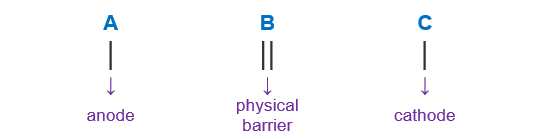# Problem: What is the shorthand notation for a galvanic cell that represents the following galvanic cell reaction?Br2(l) + 2 I-(aq) → 2 Br-(aq) + I2(s)

###### FREE Expert Solution

We’re being asked to determine the cell notation for the redox reaction shown below:

Br2(l) + 2 I-(aq) → 2 Br-(aq) + I2(s)

When writing a cell notation, we use the following format – “as easy as ABCTo determine the cell notation of the reaction, we will use the following steps:

Step 1: Separate the reaction into two half-reactions.
Step 2: Determine the anode and the cathode.
Step 3: Write the cell notation of the reaction.

81% (22 ratings)###### Problem Details

What is the shorthand notation for a galvanic cell that represents the following galvanic cell reaction?

Br2(l) + 2 I-(aq) → 2 Br-(aq) + I2(s)